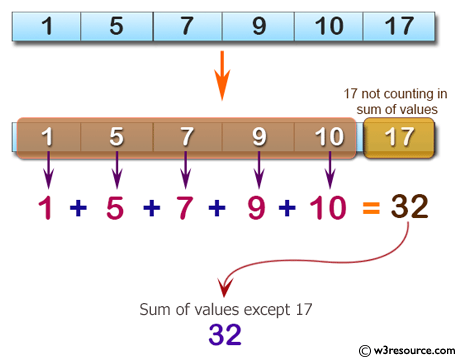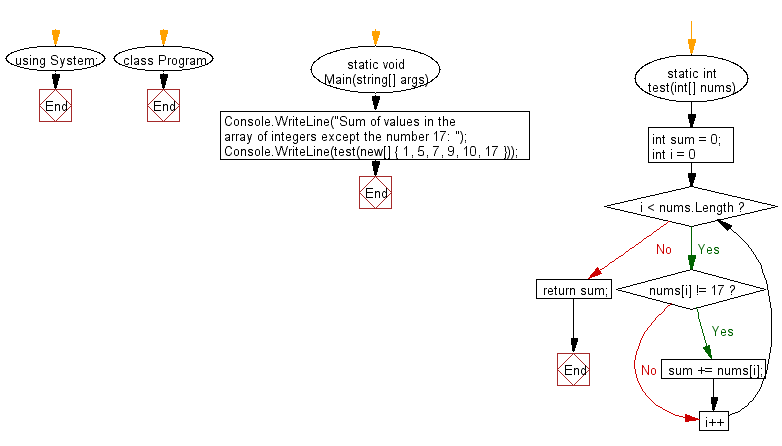﻿ C# - Sum of values in an array of integers except 17# C# Sharp Basic Algorithm Exercises: Compute the sum of values in a given array of integers except the number 17

## C# Sharp Basic Algorithm: Exercise-111 with Solution

Write a C# Sharp program to compute the sum of values in a given array of integers except the number 17. Return 0 if the given array has no integer.

Pictorial Presentation:Sample Solution:-

C# Sharp Code:

``````using System;
namespace exercises
{
class Program
{
static void Main(string[] args)
{
Console.WriteLine("Sum of values in the array of integers except the number 17: ");
Console.WriteLine(test(new[] { 1, 5, 7, 9, 10, 17 }));
}
static int test(int[] nums)
{
int sum = 0;

for (int i = 0; i < nums.Length; i++)
{
if (nums[i] != 17) sum += nums[i];
}
return sum;
}
}
}
```
```

Sample Output:

```Sum of values in the array of integers except the number 17:
32
```

Flowchart:C# Sharp Code Editor:

Improve this sample solution and post your code through Disqus

What is the difficulty level of this exercise?

Test your Programming skills with w3resource's quiz.

﻿